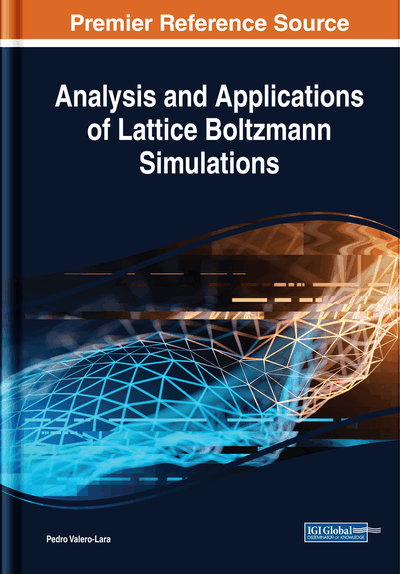# A Meshfree-Based Lattice Boltzmann Approach for Simulation of Fluid Flows Within Complex Geometries: Application of Meshfree Methods for LBM Simulations

Sonam Tanwar (University of Delhi, India)
DOI: 10.4018/978-1-5225-4760-0.ch006
Available
\$37.50
No Current Special Offers

## Abstract

This chapter develops a meshless formulation of lattice Boltzmann method for simulation of fluid flows within complex and irregular geometries. The meshless feature of proposed technique will improve the accuracy of standard lattice Boltzmann method within complicated fluid domains. Discretization of such domains itself may introduce significant numerical errors into the solution. Specifically, in phase transition or moving boundary problems, discretization of the domain is a time-consuming and complex process. In these problems, at each time step, the computational domain may change its shape and need to be re-meshed accordingly for the purpose of accuracy and stability of the solution. The author proposes to combine lattice Boltzmann method with a Galerkin meshfree technique popularly known as element-free Galerkin method in this chapter to remove the difficulties associated with traditional grid-based methods.
Chapter Preview
Top

## Introduction

Lattice Boltzmann method (LBM), originated from Ludwig Boltzmann’s kinetic theory of gases has emerged as a promising tool for fluid flow simulations besides the classical Navier-Stokes equations solvers (Begum, & Basit, 2008), (Guo et al., 2000), (Ubertini et al., 2003). The discrete Boltzmann equation is a velocity discrete form of the Boltzmann transport equation based upon microscopic model and mesoscopic kinetic theory of gases/fluids. The basic difference between standard Lattice Boltzmann method and Navier-Stokes model is that LBM is not limited to the continuum approach. Further, with a proper choice of collision operator, Navier-Stokes model can be recovered from LBM. Lattice Boltzmann method due to their simple formulation, easy implementation and substantial potential of parallel processing are suitable for flow simulations within complex/ irregular geometries (Musavia & Ashrafizaadeh, 2016), flow within porous media (Pan et al., 2006), fluid-structure interaction (FSI) problems (Kwon, 2006) and multi-phase flow problems (Kupershtokh, 2014), (Shan, & Chen, 1993).

Initially, Lattice Boltzmann method was considered to be a direct extension of Lattice Gas automata (LGA) (McNamara, & Zanetti, 1988). Later, it was shown (McNamara et al., 1995), (Sterling, & Chen, 1996) that the lattice Boltzmann equation is a special discretization of the discrete Boltzmann equation along characteristics where streaming equation becomes the exact solution of the linear advection equation. Streaming equation is commonly known as perfect shift.

However, it becomes difficult to deal with irregularly shaped geometries or curved boundaries with uniform grid structure of standard lattice Boltzmann method (Bao et al., 2008), (Chun, & Ladd, 2007), (Pan et al., 2006). This restriction is the consequence of the fact that standard lattice Boltzmann method involves discretization of velocity space and physical space coupled on a lattice (He & Luo, 1997). There have been several studies in the literature to address this drawback of the lattice Boltzmann method. There are two major approaches to extend Lattice Boltzmann method over non-uniform or unstructured grids so that it can be applied to complex problems. One is Interpolation-supplemented LBM (ISLBM), proposed by He and his colleagues (He et al., 1996). In this method, interpolation is applied at every time step in order to obtain the distribution function at the grid point. It increases the computational time and makes it computationally inefficient, especially for three-dimensional problems. The other scheme is based on the solution of a differential Lattice Boltzmann equation. The differential Lattice Boltzmann equation can further be solved by the finite volume algorithm. Succi and co-workers (Benzi et al., 1992) (Nannelli, 1992) were apparently the first to extend the technique over irregular lattice geometries by suggesting a finite volume formulation for the lattice Boltzmann equation. It was shown by Chen (Chen, 1998), that finite volume based LBM can exactly obey the conservation laws. Many authors Filippova and Hanel (Filippova, & Hanel, 1998), Dupuis and Chopard (Dupuis, & Chopard, 2003) suggested grid refinement techniques for lattice Boltzmann simulations. Although, grid-refinement algorithms may increase the efficiency of the lattice Boltzmann method, particularly in simulating strongly anisotropic flows, (e.g., high Reynolds number boundary layer flows), but still the basic problem of restriction to the uniform Cartesian grid system for standard lattice Boltzmann method remains unfixed.

## Complete Chapter List

Search this Book:
Reset